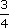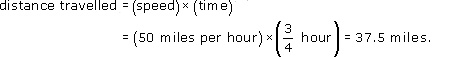Language, notation and formulas

Start this free course now. Just create an account and sign in. Enrol and complete the course for a free statement of participation or digital badge if available.

Free course

# 3.3 Units and formulas

As already noted, the units used in a formula must be consistent. For instance, in Example 9, the speed is in miles per hour, so the time travelled must be in hours and the distance travelled must be in miles. If one of the quantities is not in the correct units, then you need to change it before using the formula.

## Example 10

Suppose you have been travelling in a car at a roughly constant speed of 50 miles per hour for 45 minutes. How far would you have travelled?

You need to change the time into hours in order for the units to be consistent. Thus, 45 minutes ishour. Then, from the formula,MU120_4M6